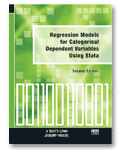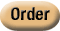»  Home »  Resources & support »  Stata news »  Regression Models for Categorical Dependent Variables Using Stata, 2nd Edition

Regression Models for Categorical Dependent Variables Using Stata, 2nd EditionRegression Models for Categorical Dependent Variables Using Stata, 2nd Edition, by J. Scott Long and Jeremy Freese, shows how to fit and interpret regression models for categorical data with Stata. Nearly 50% longer than the previous edition, the book covers new topics for fitting and interpretating models included in Stata 9, such as multinomial probit models, the stereotype logistic model, and zero-truncated count models. Many of the interpretation techniques have been updated to include interval as well as point estimates. Although regression models for categorical dependent variables are common, few texts explain how to interpret such models. Regression Models for Categorical Dependent Variables Using Stata, 2nd Edition, fills this void, showing how to fit and interpret regression models for categorical data with Stata. The authors also provide a suite of commands for hypothesis testing and model diagnostics to accompany the book. The book begins with an excellent introduction to Stata and then provides a general treatment of estimation, testing, fit, and interpretation in this class of models. Binary, ordinal, nominal, and count outcomes are covered in detail in separate chapters. The final chapter discusses how to fit and interpret models with special characteristics, such as ordinal and nominal independent variables, interaction, and nonlinear terms. One appendix discusses the syntax of the author-written commands, and a second gives details of the datasets used by the authors in the book. This book is filled with concrete examples. Because all the examples, datasets, and author-written commands are available from the authors’ website, readers can easily replicate the examples using Stata. This book is ideal for students or applied researchers who want to know how to fit and interpret models for categorical data. For further details or to order online, please visit the Stata Bookstore.